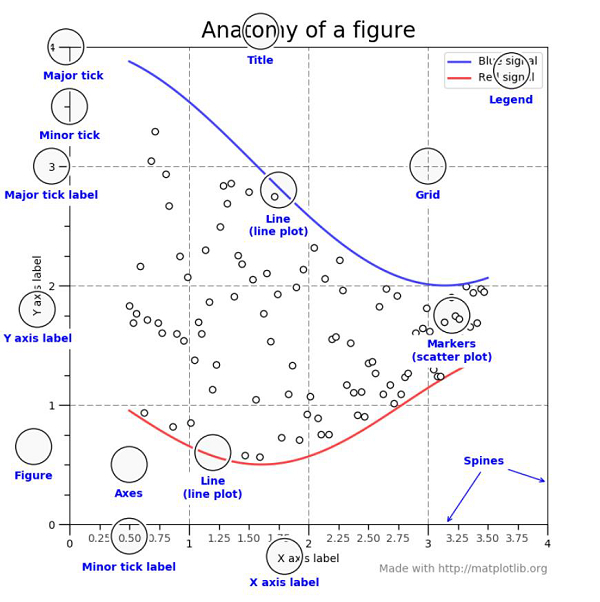# Explain about the anatomy of Matplotlib plots in Python?

Matplotlib library is built upon Numpy. It is a Python library that is used to visualize data. It is a tree−like hierarchical structure which consists of objects that makeup each of these plots.

A ’Figure’ in Matplotlib can be understood as the outermost storage for a graph. This ‘Figure’ can contains multiple ‘Axes’ objects. ‘Axes’ object is NOT the plural form of ‘Axis’ in this case.

‘Axes’ can be understood as a part of ‘Figure’, a subplot. It can be used to manipulate every part of the graph inside it. A ‘Figure’ object in an Matplotlib is a box that stores one or more ‘Axes’ objects.

Under ‘Axes’ comes the tick marks, lines, legends, and text boxes in the hierarchy. Every object in the Matplotlib can be manipulated.